Skip to main content

# Region-of-interest determination and bit-rate conversion for H.264 video transcoding

## Abstract

This paper presents a video bit-rate transcoder for baseline profile in H.264/AVC standard to fit the available channel bandwidth for the client when transmitting video bit-streams via communication channels. To maintain visual quality for low bit-rate video efficiently, this study analyzes the decoded information in the transcoder and proposes a Bayesian theorem-based region-of-interest (ROI) determination algorithm. In addition, a curve fitting scheme is employed to find the models of video bit-rate conversion. The transcoded video will conform to the target bit-rate by re-quantization according to our proposed models. After integrating the ROI detection method and the bit-rate transcoding models, the ROI-based transcoder allocates more coding bits to ROI regions and reduces the complexity of the re-encoding procedure for non-ROI regions. Hence, it not only keeps the coding quality but improves the efficiency of the video transcoding for low target bit-rates and makes the real-time transcoding more practical. Experimental results show that the proposed framework gets significantly better visual quality.

## 1. Introduction

Image and video have been widely used in our lives for many applications such as video conferencing, Internet protocol television, video phone and video surveillance, etc. To achieve these applications, an efficient video compression technology is required. H.264/AVC  video codec is one of the most popular video coding standards recently, and it overtakes MPEG-2 and other standards. After the coding process, video bit-streams are transmitted to a variety of communication channels and consumer devices. Therefore, a problem of heterogeneous communication channel can be encountered. Fortunately, the so-called transcoding technology, which can translate the data format, the bit-rate, the spatial resolution, the frame rate, and the coding standard, provides a way to solve the heterogeneous problems. The cascaded pixel-domain transcoder (CPDT)  can fully decode the bit-stream and re-encode it according to the required data format. To re-encode the video bit-streams efficiently, the transcoder is usually designed to make good use of the partially decoded information. The work in  establishes the relation of residual and coding bits using Lagrangian optimization; therefore, the appropriate motion vector at the target bit-rate could be obtained. In , the Bayesian theorem and Markov chain are utilized to model the probability of modes for the transcoding between H.264/AVC and SVC. The work in  adjusts the discrete cosine transform coefficients to decrease the ones needed to be inverted and also to create a new method to measure the distortion in this transformation. Liu and Yoo  propose a fast mode mapping method to reduce the power consumption for MPEG-2 to H.264/AVC transcoder via mode type and macroblock (MB) activity. Among all the video transcoder systems, it is especially worthy to focus on bit-rate transcoding, also known as transrating, because of the limited transmission bandwidth. As shown in Figure 1, the well-known methods to achieve bit-rate transcoding include downsizing frame resolution, frame dropping, and re-quantization of transform coefficients. Tang et al.  analyze the corresponding rate-quantization (R-Q) models for the different spatial reduction ratios (SRR). Hence, they can evaluate the proper frame size to adjust the target bit-rate. In , a parabolic motion vector re-estimation algorithm is proposed to efficiently predict the motion vectors in downscaling video for downsizing transcoder. Kapotas and Skodras  drop video frames in a compressed domain to attain suitable frame rate when the bandwidth of the network is limited. The work in  considers temporal and spatial complexities for arbitrary frame rate transcoding. Yeh et al.  analyze visual complexity and temporal coherence to propose a frame selection algorithm for temporal video transcoder. To meet the restricted network bandwidth, Kwon et al.  estimate the new quantization step (Qstep) size by using only one control parameter, which employs a simple rate control algorithm.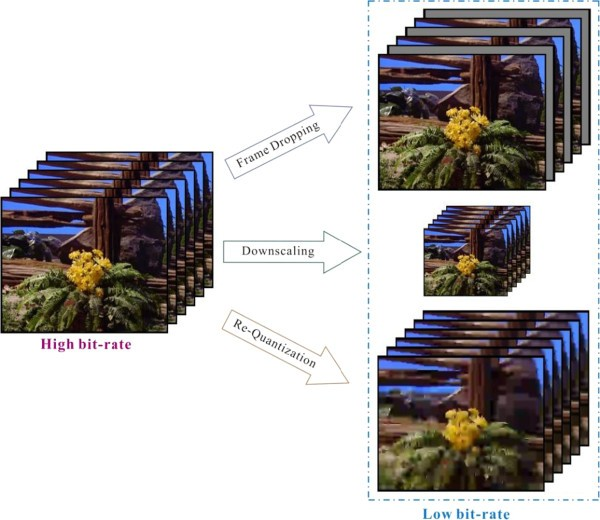Figure 1

In the applications of video processing, perceptual video coding  has obtained more and more attention due to human visual perception. Especially for the low bit-rate situation, region-of-interest (ROI)-based video coding can maintain better visual quality compared to the non-ROI video coding. According to the properties of the human visual system (HVS), the ROI areas are usually defined by skin color, contrast, video content, objects, and motion information. Liu et al.  extract moving objects in the compressed domain by considering global motion compensation, correspondence matrix, and temporal tracking. Mak and Cham  identify the background motion and then analyze the decoded motion data to complete the video object segmentation. Chi et al.  determine ROI regions through a visual rhythm feature as user attention model. Liu et al.  investigate the event detection in a compressed domain automatically. After motion trajectories are extracted, they use prediction residuals to improve the robustness of the proposed video activity detection strategy. De Bruyne et al.  estimate the reliable motion information to enhance the ROI detection based on the forward/backward projection of motion vectors (MVs) and similarity correlations. In addition, Poppe et al.  present a fast moving object detection algorithm that relies on the structure of the encoded bit-stream at the syntax level. The complicated entropy decoding to get motion information is unnecessary; thus, their method achieves high execution speeds for video surveillance applications.

Combined with a video transcoder and the idea of ROI, a compressed domain transcoder from MPEG-2 to H.264/AVC is proposed in  to reduce the computation complexity. The ROI areas are re-encoded by a closed loop transcoder for high video quality. The regions without motion vectors are defined as non-ROI and re-encoded by an open loop transcoder to speed up the transcoding process. In multipoint video conferencing, Li et al.  present a spatial adaptation ROI system to solve the limited display sizes. Kwon and Lee  propose an ROI transcoder by setting different quantization parameters (QPs) to the coded MBs via the subjective importance of different slice distributions. The center of the whole frame is the most important, and the more outer the center is, the less important it is. It can be described by a quadratic function. Although the research topic is similar to our goal, the ROI region is not precise enough in , since only the center region is defined as the ROI area. It is therefore worthy to pay more attention to the H.264/AVC ROI transcoding to reduce the complexity of the transcoder especially at the low bit-rate situation.

The proposed ROI-based video transcoder is shown in Figure 2. In this paper, by utilizing the decoded information from the front-decoder in the transcoder, we take the motion intensity and skin color information into consideration and then propose a Bayesian theorem-based ROI detection method. After estimating the ROI marcoblocks (MBs), the models of bit-rate conversion are proposed for bit-rate transcoding. Our proposed models can transcode video bit-stream to the target bit-rate by the re-quantization method and allocate more coding bits to more important areas. Furthermore, a closed-loop video transcoding system, which saves re-encoding time by reducing the processing of motion estimation in non-ROI MBs, is also proposed.Figure 2

The rest of this paper is organized as follows. Proposed ROI determination method based on Bayesian theorem describes the details of our proposed ROI detection algorithm. Proposed re-quantization models analyze the features of bit-rate conversion for baseline profile in H.264/AVC and the re-quantization models for I frames and P frames, respectively. The frameworks of our proposed ROI-based bit-rate transcoder are described in the frameworks of the proposed H.264/AVC bit-rate transcoder. Simulation results show the experimental results, and the conclusions are made finally.

## 2. Proposed ROI determination method based on Bayesian theorem

To automatically decide ROI, we employ the statistical technique to model the ROI MBs of common video content in . First of all, user-defined visual attention maps are adopted to analyze the probability distribution of the ROI. We test two sequences including Foreman and Soccer with common intermediate format (CIF 352 × 288) frame size and then analyze the relationship between the decoded data and ROI distribution. The auxiliary information includes motion intensity and skin color information. According to Bayesian theorem shown in Equations 1 and 2, we can update the ROI probability in the light of auxiliary data.

$P ROI MI = P MI ROI P ROI P MI$
(1)
$P ROI skin = P skin ROI P ROI P skin$
(2)

where P(ROI) means the prior probability of ROI before considering the auxiliary information; P(MI) and P(skin) indicate the marginal probabilities of motion intensity (MI) and skin color data, respectively. P(MI|ROI) and P(skin|ROI) represent the conditional probabilities of MI and skin color data based on ROI distribution, respectively; P(ROI|MI) and P(ROI|skin) represent the posterior probabilities of ROI given the auxiliary MI and skin color information, respectively.

It is well-known that human eyes always pay more attention to the moving regions than the background. Because of the variable block sizes adopted in H.264/AVC, we perform motion vector normalization into 4 × 4 block size to ensure fairness and then calculate the motion intensity, MI(i,j) for each MB located in (i,j) by Equation 3. To test the relationship between MI value and ROI, the average motion intensity, MIavg, for the whole frame is defined in Equation 4:

$MI i , j = 1 16 ∑ r = 0 3 ∑ s = 0 3 mvx r , s 2 + mvy r , s 2$
(3)
$MI avg = 1 h × w ∑ i = 0 h − 1 ∑ j = 0 w − 1 MI i , j$
(4)

where h and w denote the number of MBs in rows and columns of a frame, respectively; mvx and mvy are the components of motion vectors for horizontal and vertical directions, respectively. It is obvious that conditional probability increases with the larger value of motion intensity in Table 1.

However, there are still some ROI regions with lower motion. To segment ROIs accurately, we find other features more than just motion information. The human face also attracts the user's attention, and the skin color detection is used as pre-processing to efficiently extract the face region. We implement the method in  to determine skin samples by using Cb and Cr values directly. Every pixel will be labeled as skin color or not depending on whether it meets the upper and lower quadratic functions in the CbCr plane or not. Then, we discuss about the relationship between ROI and the skin color points in one MB. This additional auxiliary information can effectively solve the problem of ROIs with lower motion activity. We summarize the real and estimated probabilities of ROI by Equations 1 and 2 given the motion intensity and skin color information in Tables 1 and 2, respectively. The estimated probabilities by Equations 1 and 2 are close to the corresponding real probabilities. The flowchart of the proposed ROI detection method is shown in Figure 3. Both the motion intensity and skin color information are considered for segmenting ROI regions.Figure 3

## 3. Proposed re-quantization models

### 3.1. Analysis of video bit-rate conversion

In H.264/AVC standard, the total coding bits required for one frame contain the bits used to encode header information, motion data, and video residual as shown in Equation 5:

$R total = R header + R motion + R residual$
(5)

Header information contains the coding mode, reference frame number, transform size, etc. Compared to the whole frame coding bits, the bits used to code the header information are negligible. To improve the coding performance, H.264/AVC provides a motion vector prediction (MVP) strategy. After conducting motion estimation, the real motion vector must be subtracted by the predicted motion vector, and then only the motion vector difference is encoded. Owing to the MVP method, the bits used to code the motion data are few. Consequently, the bits used to code the video residual are the principal data in the video bit-stream. As shown in Figure 4, the higher the video bit-rate, the more residual data are retained.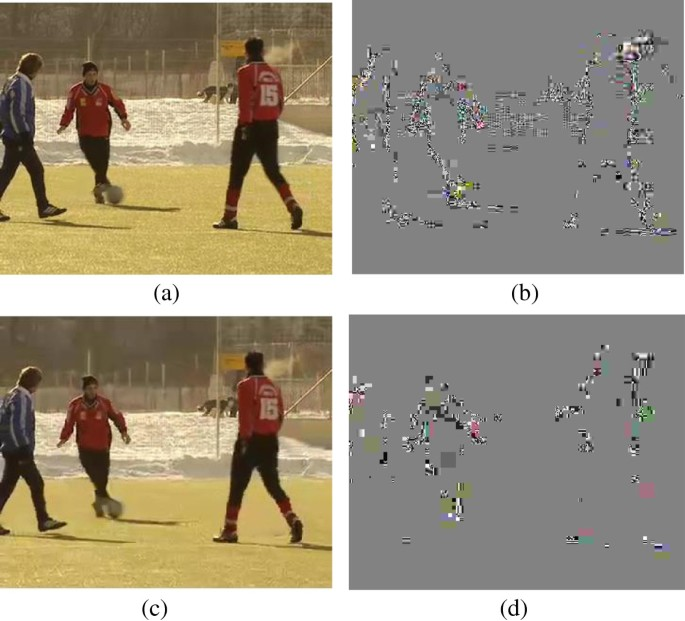Figure 4

### 3.2. Proposed re-quantization models for I and P frames

The H.264/AVC encoder performs intra/interpredictions to get the residual and then transforms this data into frequency domain as transform coefficients. Besides, QP relates to quantization operation and also influences residual data. Therefore, we take the relationship of the bit-rate, the number of non-zero transform coefficients (NZTC), and the QP into consideration to find the bit-rate conversion models.

We test six sequences in CIF (352 × 288) resolution with various characteristics including Akiyo, Hall Monitor, News, Paris, Sign Irene, and Soccer. The total number of coding frames is 100, and the group of pictures (GOP) is 15 with 1 I picture followed by 14 P pictures. Curve fitting is utilized to model the relationship between bit-rate, NZTC and QP for I frame and P frame, respectively. The accuracy of the fitting result is evaluated via R 2, also known as the coefficient of determination in regression analysis.

#### 3.2.1. I frame models

Figure 5 demonstrates the relationship of video bit-rate and NZTC for I frames. It is obvious that all of these curves have similar trends, and we can utilize Equation 6 to define the linear model. The coefficients of the corresponding video sequences are shown in Table 3.

$NZTC I = a 1 R I + b 1$
(6)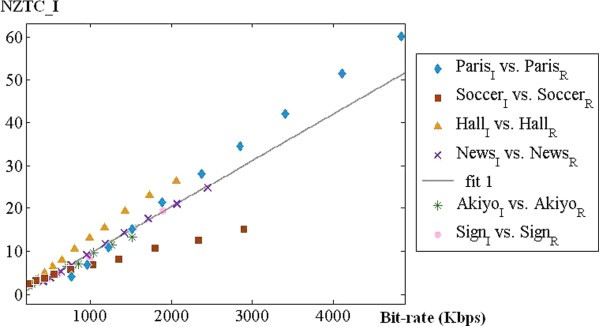Figure 5

Figure 6 exhibits the relationship of NZTC and QP data for I frames. Equation 7 illustrates the mathematical model, and Table 4 shows the coefficients of the simulated sequences. Thus, after estimating NZTC, we can get the QP value for re-quantization to reduce video bit-rate by Equation 7:

$QP I = a 2 exp b 2 NZTC I + c 2 exp d 2 NZTC I$
(7)Figure 6

#### 3.2.2. P frame models

The relationship of video bit-rate and NZTC for P frame is shown in Figure 7. Although the features of our six simulated sequences are quite different, the distribution data is similar and can be fitted accurately by Equation 8. The coefficients for different video sequences are shown in Table 5.

$NZTC P = a 3 R P 2 + b 3 R P + c 3$
(8)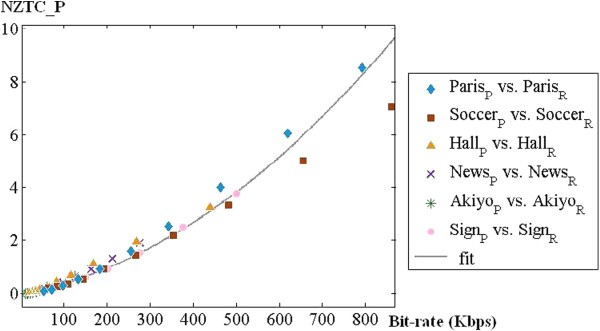Figure 7

Figure 8 shows the relationship of NZTC and QP data for P frames. Equation 9 expounds the re-quantization model so that we can accomplish the video bit-rate transcoding effectively. Table 6 shows the coefficients of our simulated sequences.

$Q P P = a 4 NZT C P b 4 + c 4$
(9)Figure 8

## 4. Frameworks of the proposed H.264/AVC bit-rate transcoder

### 4.1. Bit-rate transcoder

Equations 10 to 13 are proposed to achieve the whole processing of video bit-rate transcoding. In I frames, we can get the original number of non-zero transform coefficients (NZTCI,ori), original bit-rate (R I,ori), and original quantization parameter (QPI,ori) from the front decoder. To meet the target bit-rate (R I,tar), we propose Equation 11 to estimate target QP for re-quantization. The NZTCI,tar indicates the target number of non-zero transform coefficients shown in Equation 10. NZTCP,tar, NZTCP,ori, R P,tar, R P,ori, QPP,tar, and QPP,ori are for P frames.

$NZTC I , tar = a 1 R I , tar + b 1 a 1 R I , or i + b 1 NZTC I , ori$
(10)
$QP I , tar = a 2 exp b 2 NZTC I , tar + c 2 exp d 2 NZTC I , tar a 2 exp b 2 NZTC I , ori + c 2 exp d 2 NZTC I , ori QP I , ori$
(11)
$NZTC P , tar = a 3 R P , tar 2 + b 3 R P , tar + c 3 a 3 R P , ori 2 + b 3 R P , ori + c 3 NZTC P , ori$
(12)
$QP P , tar = a 4 NZTC P , tar b 4 a 4 NZTC P , ori b 4 QP P , ori$
(13)

Firstly, we get the original NZTC and the original bit-rate from the front decoder, and then, Equations 10 and 12 are adopted to figure the target NZTC data in the corresponding target bit-rate for I pictures and P pictures, respectively. Second, the target QP required for each MB in the rear encoder are calculated by Equations 11 and 13 for I pictures and P pictures, respectively. Therefore, the high bit-rate bit-stream can be transcoded to a low bit-rate via re-quantization method. Figure 9 refers to the architecture of our proposed bit-rate transcoding.Figure 9

### 4.2. Training procedure for model parameters

In fact, the features of various video sequences are distinctive especially for the different applications. Accordingly, the training procedure of the input sequence for its model parameters is necessary before utilizing these bit-rate conversion models. We set I frame for the first coding frame and P frame for the second frame. QP data is set to 25, 30, 35, and 40 for pre-processing coding. A curve fitting scheme is employed to find model parameters. In spite of the training procedure making a little overhead, this promotes accurate bit-rate conversion models.

### 4.3. ROI-based video bit-rate transcoder

For low bit-rate transmission of video bit-streams, the general re-quantization method diminishes the visual quality of the entire frame significantly. To solve this problem, more coding bits should be allocated in the areas with more users' interest. Hence, an automatic ROI segmentation algorithm is needed. Combined with the ROI segmentation mentioned in section 2, we propose a ROI-based bit-rate transcoder to maintain video quality in ROI MBs.

Equations 14 to 17 are proposed to allocate the limited coding bits according to the importance of each MB. Firstly, Equation 14 calculates the estimated total coding bits for the current frame after transcoding. R ori, R tar, and Bitori represent the original bit-rate, target bit-rate, and original bits for the current frame, respectively. Then, the coding bits are apportioned to MB located in (i,j) by Equation 15; w total means the weighting sum within the current frame, and w(i,j) is defined as the weighting value for MB located in (i,j). We define the weighting value as 1 if this MB is in the ROI region; otherwise, the weighting value is set to the average of conditional probabilities. After allocating coding bits for each MB by Equation 15, we multiply the Bit(i,j) with (w × h × 30) as the bit-rate for Equations 6 and 8 during the re-quantization process. Then, we adopt Equations 7 and 9 to evaluate target QP for re-quantization in the rear encoder and then transcode the video as target bit-rate. In order to reduce the coding complexity of the transcoding processing, we reuse the decoded modes for the non-ROI MBs and employ the full modes in the ROI MBs to maintain the visual quality. In addition, the H.264/AVC coding standard supports SKIP mode to improve compression efficiency, and we retain this advantage for the non-ROI areas. In short, the re-encoding time for our proposed ROI-based transcoder gets speeded up by ignoring the motion estimation and mode decision for non-ROI MBs.

$Bit total = R tar R ori Bit ori$
(14)
$Bit i , j = w i , j w total Bit total$
(15)
$w total = ∑ i = 0 h − 1 ∑ j = 0 w − 1 w i , j$
(16)
$w i , j = 1 , ( P i , j ROI | MI + P i , j ( ROI | skin ) ) / 2 , if MB i , j ∈ ROI otherwise$
(17)

Figure 9 illustrates the application of the proposed ROI determination method. It can be used in the ROI-based video transcoder to automatically extract ROI maps. The video quality in the ROI region can be better than that of the non-ROI regions for setting the different coding parameters in the encoder in Figure 9.

## 5. Simulation results

### 5.1. Setup of simulation

The proposed video bit-rate transcoder is implemented on the JM15.1  software. The computer used for our simulation has 3 GHz CPU and 3 GB RAM. The total coding frame number is set to 100 for each sequence, and the GOP is 15 with 1 I frame followed by 14 P frames. Pre-encoded bit-streams are encoded by 30 frames per second and 1 megabits per second (Mbps). The search range is set to +/−32, and the number of reference frames is set to one for motion estimation. The 8 × 8 transform size is disabled. We adopt the peak signal-to-noise ratio (PSNR) and structural similarity (SSIM)  for the quality evaluation. The SSIM value used to measure the structural distortion is between −1 and 1, and it is a popular method owing to its high correlation with HVS.

### 5.2. Simulation results of ROI detection

The decoded 49th frame in the Carphone sequence and the ROI detection result by  are shown in Figure 10a,b. Figure 10c demonstrates the ROI extraction without skin color data. This method could not extract the human face accurately. Figure 10d improves the segmentation results according to the skin color information. The different thresholds for T MI and T skin are shown in Figure 10e.Figure 10

Figure 11a, b illustrates MI data and skin data, respectively. The definitions of gray color are shown in Table 7. From Figure 11a, we can observe that the motion information could be inconsistent in the whole frame. Therefore, the ROI determination result will be more precise with both MI information and skin data.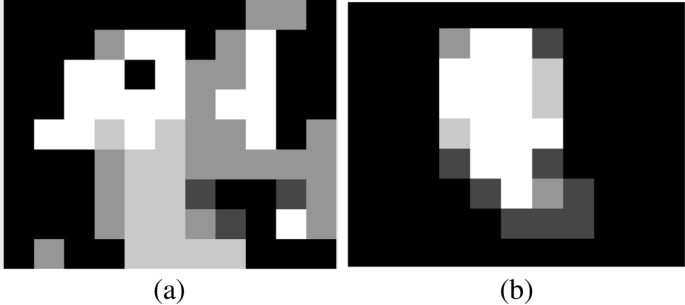Figure 11

### 5.3. Simulation results of the proposed ROI-based bit-rate transcoding

We test three sequences including Akiyo, Foreman, and News in CIF (352 × 288) format. Tables 8, 9, and 10 express the experimental results of the bit-rate data, coding time, and ROI PSNR comparison, respectively. We transcode simulated sequences from 1 Mbps to 220 Kbps, 900 Kbps, and 390 Kbps. The CPDT and proposed ROI-based transcoder represent the results produced by the cascaded pixel domain transcoder and the proposed ROI-based transcoder, respectively. From Tables 8, 9, and 10, it is obvious that the CPDT method controls the bit-rate accurately but consumes much coding time because of fully decoding and encoding the video sequences. The proposed ROI-based transcoder reduces re-encoding time significantly and saves computational time at least by 47.52%. The proposed method also provides better visual quality in ROI regions ranging from 1.35 to 3.36 dB.

Figures 12, 13, and 14 demonstrate the comparison results of the subjective qualities for Akiyo, Foreman, and News sequences, respectively. We can observe that our ROI-based transcoder outperforms the CPDT method in both PSNR and SSIM measurements and the subjective quality. The proposed ROI-based scheme takes advantage of the human visual system and also reduces the computational complexity of the H.264 video transcoder dramatically.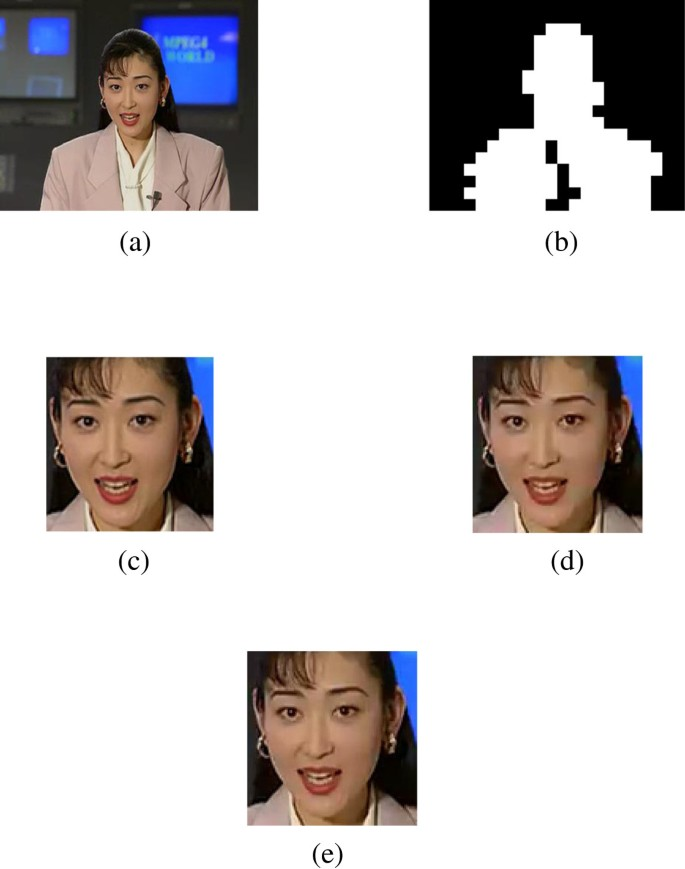Figure 12Figure 13Figure 14

## 6. Conclusions

In this paper, a ROI-based video transcoder is proposed. Firstly, the Bayesian theorem is applied to automatically determine the ROI region for video transcoding. The region with moving foreground objects is segmented by decoding pre-encoded motion information from the input of the video transcoder. The skin color information is used to further find out the region of human attention. Second, a model-based video bit-rate transcoder is presented for baseline profile in H.264/AVC to meet the available communication bandwidth. We analyze the total coding bit-rate, NZTC, and QP data in the H.264/AVC encoder to get our proposed models and reallocate the bits of each macroblock in consideration of ROI for bit-rate conversion. By virtue of the estimated target QP by the proposed I frame and P frame models, the simulated results show that the transcoded bit-stream conforms to the target bit-rate via the re-quantization method. Furthermore, this paper also proposes a ROI-based video transcoder to maintain visual quality in the region of user's interest for low bit-rate transmission. More coding bits are allocated in ROI MBs, and full coding modes are employed to ensure visual quality. We utilize the SKIP and the decoded mode for motion estimation to reduce coding complexity on non-ROI MBs. Experimental results show that the proposed video bit-rate transcoder provides efficient video transcoding, accurate performance, and better video quality. In the future, the models of the total coding bit-rate, NZTC, and QP data for B frames will be investigated for the applications of high profile H.264/AVC video standard.

## References

1. 1.

Kwon SK, Tamhankar A, Rao KR: Overview of H.264/MPEG-f part 10. J. Visual Commun. Image Rep. 2006, 17: 186-216. 10.1016/j.jvcir.2005.05.010

2. 2.

Xin J, Lin CW, Sun MT: Digital video transcoding. Proceedings of the IEEE 2005, 93(1):84-97.

3. 3.

Yeh CH, Fan Jiang SJ, Chen TC, Chen MJ: Motion vector composition through Lagrangian optimization for arbitrary frame-size video transcoding. Optical Engineering 2012, 51(4):047401. 10.1117/1.OE.51.4.047401

4. 4.

Yeh CH, Tseng WY, Wu ST: Mode decision acceleration for H.264/AVC to SVC temporal video transcoding. EURASIP J. Adv. Signal Process 2012., 204:

5. 5.

Xin J, Vetro A, Sun H, Su Y: Efficient MPEG-2 to H.264/AVC transcoding of intra-coded video. EURASIP J. Adv. Signal Process 2007, 2007: 075310. 10.1155/2007/75310

6. 6.

Liu X, Yoo KY: Fast interframe mode decision algorithm based on mode mapping and MB activity for MPEG-2 to H.264/AVC transcoding. J. Visual Commun. Image Rep 2010, 21: 155-166. 10.1016/j.jvcir.2009.05.002

7. 7.

Tang Q, Mansour H, Nasiopoulos P, Ward R: Bit-rate estimation for bit-rate reduction H.264/AVC video transcoding in wireless networks. In Proceedings of International Symposium on Wireless Pervasive Computing. Santorini; 7–9 May 2008:464-467.

8. 8.

Yeh CH, Chen YH, Chi MC, Chen MJ: Parabolic motion-vector re-estimation algorithm for compressed video downscaling. J. Signal Process. Syst. 2010, 61(3):375-386. 10.1007/s11265-010-0455-z

9. 9.

Kapotas SK, Skodras AN: Bit rate transcoding of H.264 encoded movies by dropping frames in the compressed domain. IEEE Trans. Consum. Electron. 2010, 56(3):1593-1601.

10. 10.

Hsu CT, Yeh CH, Chen CY, Chen MJ: Arbitrary frame rate transcoding through temporal and spatial complexity. IEEE Trans. Broadcast. 2009, 55(4):767-775.

11. 11.

Yeh CH, Fan Jiang SJ, Lin CY, Chen MJ: Temporal video transcoding based on frame complexity analysis for mobile video communication. IEEE Trans. Broadcast 2013, 59(1):38-46.

12. 12.

Kwon SK, Kin SW, Lee JH, Lee JM: An adaptive transcoding method for H.264 video coding. IJCSNS 2008, 8(12):154-160.

13. 13.

Chen Z, Lin W, Ngan KN: Perceptual video coding: challenges and approaches. Proceedings of the IEEE International Conference on Multimedia Expo, Suntec City, 19–23 Jul 2010 784-789.

14. 14.

Liu Z, Lu Y, Zhang Z: Real-time spatiotemporal segmentation of video objects in the H.264 compressed domain. J. Visual Commun. Image Rep. 2007, 18: 275-290. 10.1016/j.jvcir.2007.02.002

15. 15.

Mak CM, Cham WK: Real-time video object segmentation in H.264 compressed domain. IET Image Processing. 2009, 3(5):272-285. 10.1049/iet-ipr.2008.0093

16. 16.

Chi MC, Yeh CH, Chen MJ: Robust region-of-interest determination based on user attention model through visual rhythm analysis. IEEE Trans. Circuits Syst. Video Technol 2009, 19(7):1025-1038.

17. 17.

Liu H, Sun MT, Wu RC, Yu SS: Automatic video activity detection using compressed domain motion trajectories for H.264 videos. J. Visual Commun. Image Rep. 2011, 22: 432-439. 10.1016/j.jvcir.2011.03.010

18. 18.

De Bruyne S, Poppe C, Verstockt S, Lambert P, Van de Walle R: Estimating motion reliability to improve moving object detection in the H.264/AVC domain. Proceedings of IEEE International Conference on Multimedia and Expo, New York, 28 Jun-3 July 2009 330-333.

19. 19.

Poppe C, De Bruyne S, Paridaens T, Lambert P, Van de Walle R: Moving object detection in the H.264/AVC compressed domain for video surveillance applications. J. Visual Commun. Image Rep 2009, 20: 428-437. 10.1016/j.jvcir.2009.05.001

20. 20.

Xie R, Yu S: Region-of-interest based video transcoding from MPEG-2 to H.264 in the compressed domain. Optical Engineering 2008, 47(9):097001–-1-097001-7.

21. 21.

Li H, Wang Y, Chen CW: An attention-information based spatial adaptation framework for browsing videos via mobile devices. EURASIP J. Adv. Signal Process 2007, 2007: 1-12.

22. 22.

Kwon SK, Lee HY: A transcoding method for improving the subjective picture quality. IJCSNS 2009, 9(1):135-138.

23. 23.

Huang SF, Chen MJ, Li MS: Region-of-interest segmentation based on Bayesian theorem for H.264 video transcoding. In Proceedings of Visual Communications and Image Processing Conference. Tainan; 6–9 Nov 2011.

24. 24.

Chi MC, Chen MJ, Yeh CH, Jhu JA: Region-of-interest video coding based on rate and distortion variations for H.263+. Signal Process: Image Commun. 2008, 23(2):127-142. 10.1016/j.image.2007.12.001

25. 25.

Joint Video Team software JM http://iphome.hhi.de/suehring/tml/download/

26. 26.

Wang Z, Bovik AC, Sheikh HR, Simoncelli EP: Image quality assessment: form error visibility to structural similarity. IEEE Trans. Image Processing 2004, 13(4):600-612. 10.1109/TIP.2003.819861

Download references

## Author information

Authors

### Corresponding author

Correspondence to Mei-Juan Chen.

## Additional information

### Competing interests

The authors declare that they have no competing interests.

## Authors’ original submitted files for images

Below are the links to the authors’ original submitted files for images.

## Rights and permissions

Open Access This article is distributed under the terms of the Creative Commons Attribution 2.0 International License ( https://creativecommons.org/licenses/by/2.0 ), which permits unrestricted use, distribution, and reproduction in any medium, provided the original work is properly cited.

Reprints and Permissions

## About this article

### Cite this article

Huang, SF., Chen, MJ., Tai, KH. et al. Region-of-interest determination and bit-rate conversion for H.264 video transcoding. EURASIP J. Adv. Signal Process. 2013, 112 (2013). https://doi.org/10.1186/1687-6180-2013-112

Download citation

• Received:

• Accepted:

• Published:

### Keywords

• Video transcoding
• Region-of-interest
• Re-quantization
• Motion vector